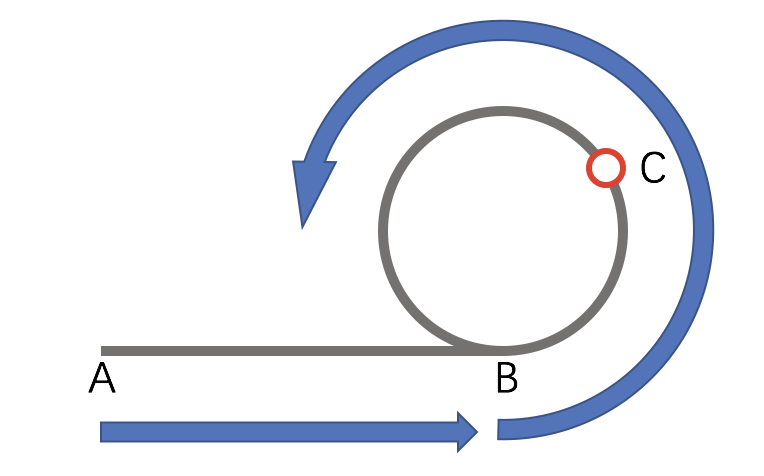Given an array _nums_ containing _n_ + 1 integers where each integer is between 1 and _n_ (inclusive), prove that at least one duplicate number must exist. Assume that there is only one duplicate number, find the duplicate one.

Example 1:

Example 2:

Note:

1. You must not modify the array (assume the array is read only).
2. You must use only constant, _O_(1) extra space.
3. Your runtime complexity should be less than _O_(n2).
4. There is only one duplicate number in the array, but it could be repeated more than once.1. $a+xc$的意义就是，从 A 点出发，在 B 点进入循环，并经过$x$圈又回到 B 点
2. $(N-2n-1+x)c+c-b$的意思则是：从 C 点继续向后走，走过$c-b$的长度后，回到 B 点，又经过$(N-2n-1+x)$圈回到 B 点
3. 上述两者相同的意思是，此时指针又会共同指向 B 点，那么我们就找到了进入循环的点。

## 关于快慢指针的应用

• 单向链表是否存在循环：只要快指针和慢指针相遇，则一定存在环
• 寻找无环链表的中位数：当快指针指向链尾时，慢指针指向链中
• 找链表中环的入口点：如上所述
• 两个单向无环链表是否相交：将其中一个链表首尾相连，另一个链表开始进行快慢指针遍历，就转化成了第一个问题